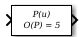Polynomial

Perform evaluation of polynomial coefficients on input values

• Library:

•Description

The Polynomial block evaluates P(u) at each time step for the input u. You define a set of polynomial coefficients in the form that the MATLAB® polyval command accepts.

Ports

Input

expand all

Value at which to evaluate the polynomial P(u).

Data Types: single | double

Output

expand all

Value of the polynomial P(u) evaluated at the input signal.

Data Types: single | double

Parameters

expand all

Specify polynomial coefficients in MATLAB polyval form. The first coefficient corresponds to xN and the remaining coefficients correspond to decreasing orders of x. The last coefficient represents the constant for the polynomial.

Programmatic Use

 Block Parameter: coefs Type: real array Default: [ +2.081618890e-019, -1.441693666e-014, +4.719686976e-010, -8.536869453e-006, +1.621573104e-001, -8.087801117e+001 ]

Block Characteristics

 Data Types double | single Direct Feedthrough yes Multidimensional Signals no Variable-Size Signals no Zero-Crossing Detection no